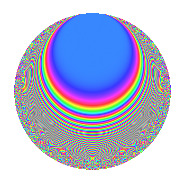# Properties

 Label 335.2.cLevel 335 Weight 2 Character orbit c Rep. character $$\chi_{335}(269,\cdot)$$ Character field $$\Q$$ Dimension 32 Newform subspaces 1 Sturm bound 68 Trace bound 0

# Related objects

## Defining parameters

 Level: $$N$$ = $$335 = 5 \cdot 67$$ Weight: $$k$$ = $$2$$ Character orbit: $$[\chi]$$ = 335.c (of order $$2$$ and degree $$1$$) Character conductor: $$\operatorname{cond}(\chi)$$ = $$5$$ Character field: $$\Q$$ Newform subspaces: $$1$$ Sturm bound: $$68$$ Trace bound: $$0$$

## Dimensions

The following table gives the dimensions of various subspaces of $$M_{2}(335, [\chi])$$.

Total New Old
Modular forms 36 32 4
Cusp forms 32 32 0
Eisenstein series 4 0 4

## Trace form

 $$32q - 34q^{4} - 32q^{9} + O(q^{10})$$ $$32q - 34q^{4} - 32q^{9} + 8q^{10} + 4q^{11} - 4q^{14} + 2q^{15} + 30q^{16} - 4q^{19} - 18q^{20} - 16q^{21} + 28q^{24} - 4q^{25} + 8q^{26} + 12q^{29} - 6q^{30} - 28q^{34} - 2q^{35} + 50q^{36} + 24q^{39} - 2q^{40} + 16q^{41} + 8q^{44} - 16q^{45} - 32q^{46} + 8q^{49} - 12q^{50} + 12q^{51} - 4q^{54} - 52q^{55} + 4q^{56} + 8q^{59} - 6q^{60} - 8q^{61} - 66q^{64} - 14q^{65} + 52q^{66} - 40q^{69} + 34q^{70} - 8q^{71} - 12q^{74} - 18q^{75} + 52q^{76} + 36q^{79} + 44q^{80} + 8q^{81} - 14q^{84} + 18q^{85} + 6q^{86} + 52q^{89} - 4q^{90} + 12q^{91} - 20q^{95} - 86q^{96} - 16q^{99} + O(q^{100})$$

## Decomposition of $$S_{2}^{\mathrm{new}}(335, [\chi])$$ into newform subspaces

Label Dim. $$A$$ Field CM Traces $q$-expansion
$$a_2$$ $$a_3$$ $$a_5$$ $$a_7$$
335.2.c.a $$32$$ $$2.675$$ None $$0$$ $$0$$ $$0$$ $$0$$

## Hecke Characteristic Polynomials

There are no characteristic polynomials of Hecke operators in the database